Courses

# Push Down Automata: CFL & DCFL (Advance Level) - 1

## 15 Questions MCQ Test Question Bank for GATE Computer Science Engineering | Push Down Automata: CFL & DCFL (Advance Level) - 1

Description
This mock test of Push Down Automata: CFL & DCFL (Advance Level) - 1 for Computer Science Engineering (CSE) helps you for every Computer Science Engineering (CSE) entrance exam. This contains 15 Multiple Choice Questions for Computer Science Engineering (CSE) Push Down Automata: CFL & DCFL (Advance Level) - 1 (mcq) to study with solutions a complete question bank. The solved questions answers in this Push Down Automata: CFL & DCFL (Advance Level) - 1 quiz give you a good mix of easy questions and tough questions. Computer Science Engineering (CSE) students definitely take this Push Down Automata: CFL & DCFL (Advance Level) - 1 exercise for a better result in the exam. You can find other Push Down Automata: CFL & DCFL (Advance Level) - 1 extra questions, long questions & short questions for Computer Science Engineering (CSE) on EduRev as well by searching above.
QUESTION: 1

### Which of the following is false?

Solution:

If a language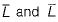both recursively enumerable, then both L and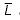are recursive.

QUESTION: 2

### For the grammar given below, what is the equivalent CFG without useless symbols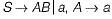Solution:

Since in grammar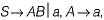is useless and so is A. Hence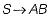is useless. Therefore the equivalent CFG is  S → a.

QUESTION: 3

### For the given grammar below, what is the equivalent CFG without ∈ -productions?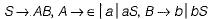Solution:

For the given grammar: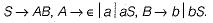Only A is nullable. Hence replacing and adding at each and every place with A with ∈ we have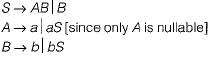QUESTION: 4

What is the equivalent CFL for the following CFG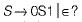Solution:

The given CFG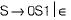always produce equal number of 0'S followed by equal number of 1’s. It also contains the ∈. Hence the equivalent CFL is nothing but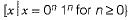QUESTION: 5

For the given grammar below, what is the equivalent CFG without ∈-productions?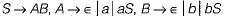Solution:

Since the CFG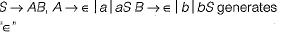So L(G), itself contain the ‘∈’ and it is not possible from a CFG to remove ‘∈ ’ production if ‘∈’ is part of the language.

QUESTION: 6

What is the equivalent CFL for the following CFG?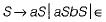Solution:

The given CFG: S → aS|aSdS|∈
denotes each prefix of x has atleast as many a’s as b’s.

QUESTION: 7

Which of the following languages can’t be accepted by a deterministic PDA?

Solution:

Deterministic PDA can accept
(b) The set of all strings of balanced parenthesis.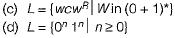In each of the above the PDA can deterministically separate the push and pop operations,
But it cannot do the same in case (a), since it has to guess middle of palindrome, In DPDA we can’t do guesing, NPDA is required for this.

QUESTION: 8

Which of the following is a CFL?

Solution: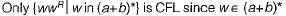and we cannot determine the middle of the wwR i.e. we cannot determine the end of ' w" and start of wR. In all other options, there are more than one comparison.

QUESTION: 9

Which language does the following PDA accept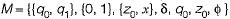and b is given by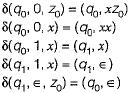Solution: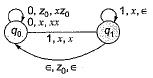So, strain at least contain 011, push 0, skip 1st 1 then POP 0 for second 1, the accept by ∈, z0, t transition.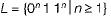QUESTION: 10

Which language does M accept if the following transition is added?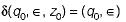Solution: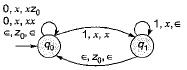Since ∈ can also be accepted, 0n push then skip 1st 1, then pop all 0 for 1n and accept by transition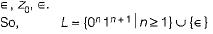QUESTION: 11

Consider the following set of languages: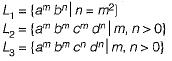Which of the above language is not context free?

Solution: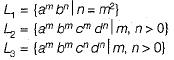In L1 n = m2 cannot be checkes in stack as m2 is a nonlinear function.
Hence it is not CFL.
L2 requires 2 comparisons on number as itself
⇒ Number of a’s = number of b’s and number of c’s. This cannot be done by single stack
L3 requires 2 comparisons, but only one at a time is required.
⇒ Number of a’s = number of b’s and number of c’s = number of d/’s. This can be done in single stack.

QUESTION: 12

L has the following grammar: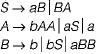L2 has the following grammar:
S → Sba\a
Which of the following statement is true about?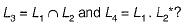Solution:

L1 and L2 are context free languages. We can see that L2 is in fact a regular language having regular expression a (ba)*.
L1 is a language containing strings having equal number of a’s and b’s.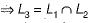is a context free language,
∴ class of context free languages is closed under concatenation and kleene closure. There fore L4 = L1 . L2* is a context free.

QUESTION: 13

Which of the following is not true?

Solution:

• Every LL grammar must be unambiguous but every unambiguous need not be LL grammar.
• Ambiguity in language starts from CFL. So, DCFL cannot be inherently ambiguous.
• if G is an LR(K) grammar, then L{G) is a DCFL.
• Every CFL’s which are not DCFL need not be ambiguous.

QUESTION: 14

Consider the grammar consisting of 7 productions:-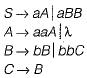After elimination of Unit, useless and λ-productions, how may production remain in the resulting grammar?

Solution:

B and C are useless, since they are not generating any terminal.
Therefore the remaining productions left after removing useless, unit and λ-productions.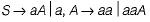QUESTION: 15

Consider the CFG G( \/, T, P, S) with the following production: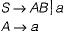Let CFG G' is an equivalent CFG with no useless symbols. How many minimum production will be there in G'?

Solution: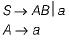Here S → AB is useless because of symbol B.
Hence the production A → a is also useless. There fore we are left with only S → a.